# L-systems

(back to contents)

In 1968 a biologist, Aristid Lindenmayer, introduced a variant of formal grammars - the Lindenmayer system (or simply L-system). L-system formalism consists of an axiom and derivation rules. Each word (or symbol) in the axiom is rewritten according to rules, thus obtaining a production. Productions then can be rewritten again and again any number of times.```(with-graphics (set-axiom (turn(-5) A)) (add-rule (A -> F(50) turn(10) F(50) turn(-10) A)) ```

To add an L-system rule, `add-rule` macro must be used within `with-graphics`. The argument to this macro is a rule which looks something like `(S0 -> S1 S2 S3)`. That means that each symbol `S0` in the current production will be replaced with symbols `S1 S2 S3` in the next production. Unfortunately the above example draws nothing because turtle starts interpreting the axiom right away. It turns -5 degrees and skips symbol `A` because there is no drawing command associated with it.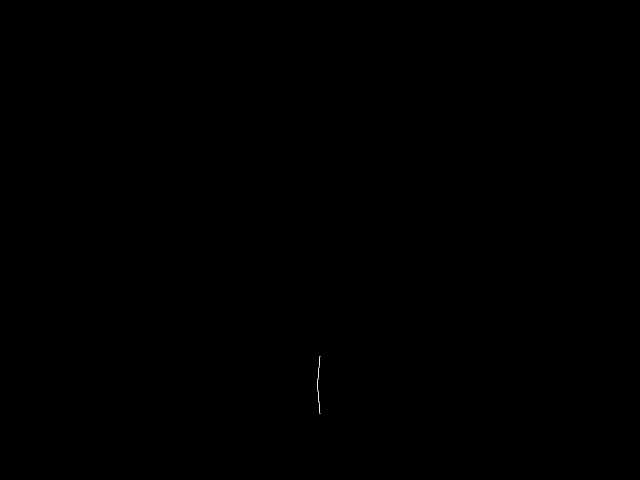```(with-graphics (iteration-count 1) (set-axiom (turn(-5) A)) (add-rule (A -> F(50) turn(10) F(50) turn(-10) A)) ```

To get the program to rewrite the L-system the desired number of times, `iteration-count` function must be used. If `iteration-count` is not called, iteration count defaults to zero, which is why nothing was rewritten in the previous example. However, in this example:

`turn(-5) A`

is rewritten once:

`turn(-5) F(50) turn(10) F(50) turn(-10) A`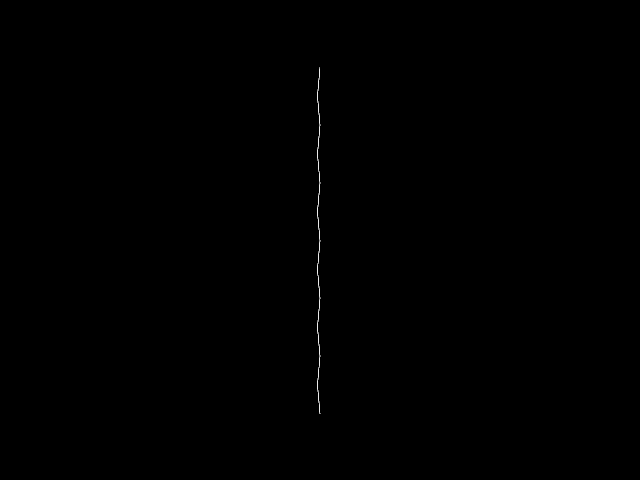```(with-graphics (iteration-count 6) (set-axiom (turn(-5) A)) (add-rule (A -> F(50) turn(10) F(50) turn(-10) A)) ```

This example is exactly like the previous one except for the iteration count:

axiom:
`turn(-5) A`

1st production:
`turn(-5) F(50) turn(10) F(50) turn(-10) A`

2nd production:
```turn(-5) F(50) turn(10) F(50) turn(-10) F(50) turn(10) F(50) turn(-10) A```

3rd production:
```turn(-5) F(50) turn(10) F(50) turn(-10) F(50) turn(10) F(50) turn(-10) F(50) turn(10) F(50) turn(-10) A```

...

6th production:
```turn(-5) F(50) turn(10) F(50) turn(-10) F(50) turn(10) F(50) turn(-10) F(50) turn(10) F(50) turn(-10)          F(50) turn(10) F(50) turn(-10) F(50) turn(10) F(50) turn(-10) F(50) turn(10) F(50) turn(-10) A```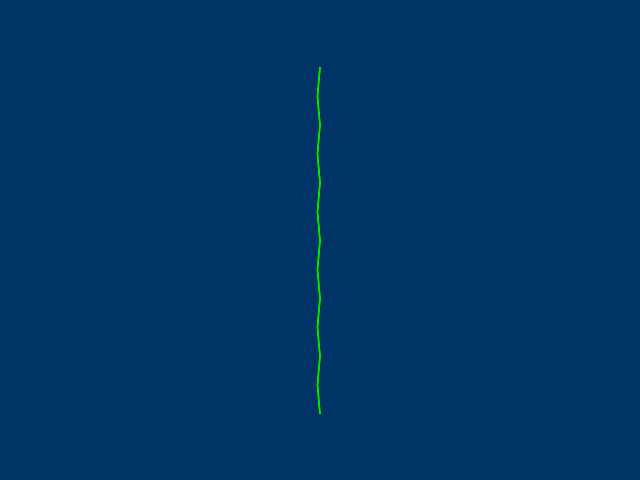```(defparameter *green* (create-color 0.0 1.0 0.0)) (with-graphics (iteration-count 6) (set-axiom (radius(2) color(*green*) turn(-5) A)) (add-rule (A -> F(50) turn(10) F(50) turn(-10) A)) (set-background-color (create-color 0.0 0.2 0.4))) ```

In this example some features that were described in the previous chapter have been added.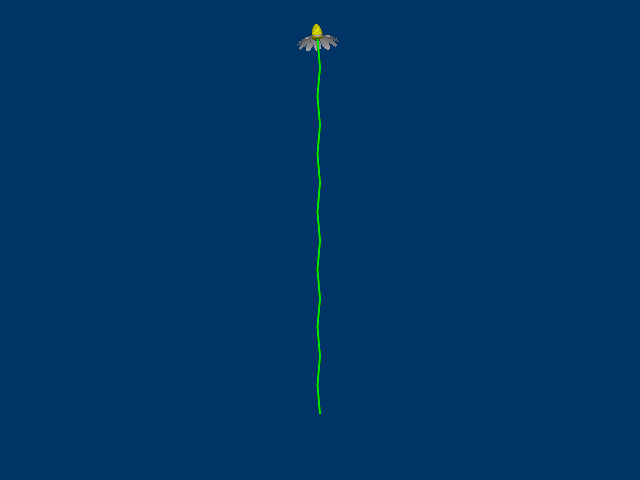```(defparameter *green* (create-color 0.0 1.0 0.0)) (with-graphics (iteration-count 6) (set-axiom (radius(2) color(*green*) turn(-5) A F(50) obj("blossom" 10.0))) (add-rule (A -> F(50) turn(10) F(50) turn(-10) A)) (set-background-color (create-color 0.0 0.2 0.4)) (read-wavefront "blossom.obj" "blossom.png")) ```

It is convenient to design an L-system so that rules have semantic meaning. In this example, rule `A` represents the flower's stem. Everything that comes before this rule will form the base of the plant, and everything that comes after it is at the apex of the plant (e.g., the "blossom" object).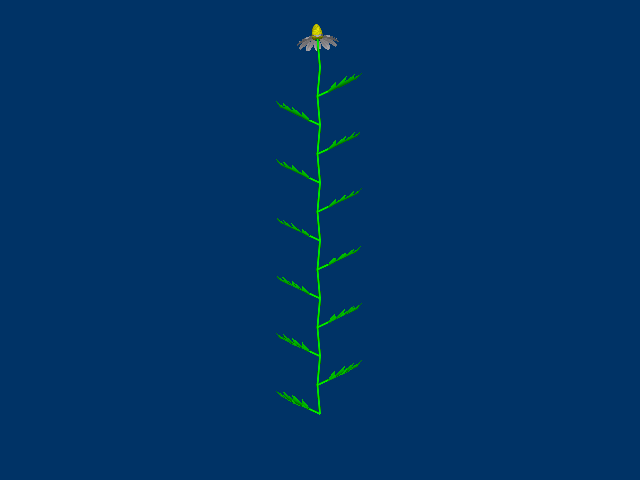```(defparameter *green* (create-color 0.0 1.0 0.0)) (with-graphics (iteration-count 6) (set-axiom (radius(2) color(*green*) turn(-5) A F(50) obj("blossom" 10.0))) (add-rule (A -> [ roll(180) L ] F(50) turn(10) [ L ] F(50) turn(-10) A)) (set-background-color (create-color 0.0 0.2 0.4)) (read-wavefront "blossom.obj" "blossom.png") (add-macro (L -> turn(60) F(20) obj("leaf" 10.0 *green*))) (read-wavefront "leaf.obj")) ```

`add-macro` is used to help add leaves to the plant. Its syntax is very similar to `add-rule`, except that `add-macro` can not recursively expand to itself. L-system macros are expanded into rules prior to rewriting. For that reason `(L -> L)` is a bad macro, because semantically it will infinitely expand to itself, but practically LISP will say that "The function L is undefined". Here is an example that illustrates the differences between `add-rule` and `add-macro`:

 ```(add-macro (B -> A)) (add-rule (A -> B A)) ``` is the same as: `(add-rule (A -> A A))` and after 3 steps expands `A` to: `(A A A A A A A A)`

while

 ```(add-rule (B -> A)) (add-rule (A -> B A)) ``` after 3 steps expands `A` to: `(B A A B A)`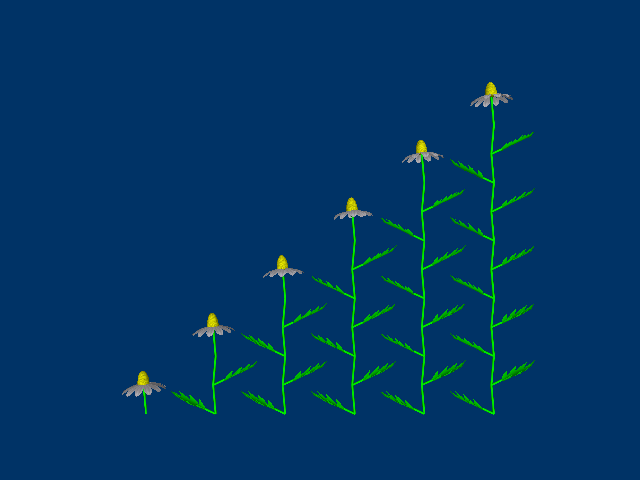```(defparameter *green* (create-color 0.0 1.0 0.0)) (with-graphics (iteration-count 7) (set-axiom (radius(2) color(*green*) move((create-point 300.0 0.0 0.0)) X)) (add-rule (A -> [ roll(180) L ] F(50) turn(10) [ L ] F(50) turn(-10) A)) (set-background-color (create-color 0.0 0.2 0.4)) (read-wavefront "blossom.obj" "blossom.png") (add-macro (L -> turn(60) F(20) obj("leaf" 10.0 *green*))) (read-wavefront "leaf.obj") (add-rule (P -> A F(50) obj("blossom" 10.0) )) (add-rule (X -> [ turn(-5) P ] turn(-90) jump(120) turn(90) X))) ```

To illustrate how an L-system is rewritten, the last example introduces two new rules: `P` which semantically means "plant", and `X` which semantically means "a row of plants". It also introduces two new turtle commands. `jump` moves turtle forward in the same manner as `forward`, except that `jump` does not cause turtle to leave a trace. The command `move` takes a point structure as argument and "teleports" turtle to that specific location in absolute coordinates. Bottom middle of the screen where turtle starts its life has coordinates `(create-point 0.0 0.0 0.0)`. Moving turtle to coordinates `(create-point 300.0 0.0 0.0)` moves turtle 300.0 units along the x axis to the right where the base of the longest plant is.

(back to contents)